### IMO Shortlist 2006 problem N3

Kvaliteta:
Avg: 0,0
Težina:
Avg: 6,5
We define a sequence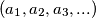$\left(a_{1},a_{2},a_{3},...\right)$ by setting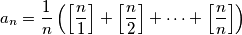for every positive integer$n$. Hereby, for every real$x$, we denote by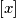$\left[x\right]$ the integral part of$x$ (this is the greatest integer which is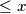$\leq x$).

a) Prove that there is an infinite number of positive integers$n$ such that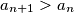$a_{n + 1} > a_{n}$.
b) Prove that there is an infinite number of positive integers$n$ such that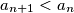$a_{n + 1} < a_{n}$.
Izvor: Međunarodna matematička olimpijada, shortlist 2006